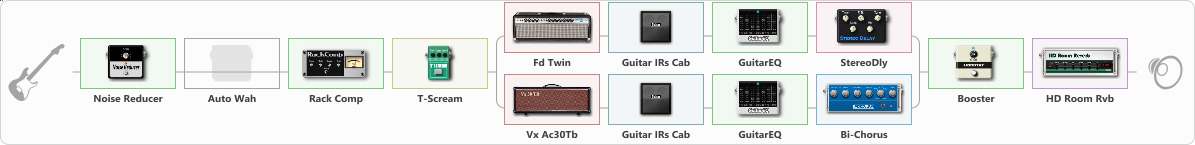# Temple Of The King Solo Clean

Discussion in 'ToneLib-GFX presets' started by d4nte1988, Oct 9, 2022.

1. Temple Of The King Solo Clean

Preset name: Temple Of The King_Clean

Effects chain:Effect: "Noise Reducer" (Dynamics / Filter), active - "yes"
{
"Sens" = 76
"Mode" = Hard
}

Effect: "Auto Wah" (Dynamics / Filter), active - "no"
{
"Sense" = 74
"Polarity" = Up
"Attack" = 17
"Style" = V848
}

Effect: "Rack Comp" (Dynamics / Filter), active - "yes"
{
"Threshold (dB)" = 2
"Ratio" = 5
"Attack" = Slow
"Release (ms)" = 250
"Knee" = 25
"Level (dB)" = 0
}

Effect: "T-Scream" (Овердрайв / Дисторшн), active - "yes"
{
"Drive" = 1
"Tone" = 80
"Level" = 79
}

Effect: "Splitter" (Dynamics / Filter)
{
"A-Bypass" = Off
"A-Pan" = -26
"A-Level" = 76
"B-Bypass" = Off
"B-Pan" = 51
"B-Level" = 53
"Width" = 0

'A' branch:
{

Effect: "Fd Twin" (Симуляторы усилителей), active - "yes"
{
"Gain" = 65
"Bass" = 50
"Middle" = 50
"Treble" = 68
"Presence" = 79
"Master" = 76
"Level (dB)" = 0
}

Effect: "Guitar IRs Cab" (Кабинеты), active - "yes"
{
"Model" = Marshall 1960A (4x12")
"Mic Position" = Middle
"Mic Distance" = Middle
"Low Cut (Hz)" = 54
"Hi Cut (kHz)" = 8.0
"Mix" = 100
"Level (dB)" = 5
}

Effect: "GuitarEQ" (Dynamics / Filter), active - "yes"
{
"160 Hz" = 2
"400 Hz" = 2
"800 Hz" = 2
"1.6 kHz" = -3
"3.2 kHz" = -2
"6.4 kHz" = -5
"12 kHz" = -15
"Level (dB)" = 0
}

Effect: "StereoDly" (Дилей), active - "yes"
{
"Time" = 689
"Feedback" = 41
"Tone" = 76
"Sens" = 75
"Mix" = 68
}
}
'B' branch:
{

Effect: "Vx Ac30Tb" (Симуляторы усилителей), active - "yes"
{
"Gain" = 100
"Bass" = 50
"Middle" = 61
"Treble" = 72
"Presence" = 75
"Master" = 74
"Level (dB)" = 0
}

Effect: "Guitar IRs Cab" (Кабинеты), active - "yes"
{
"Model" = Marshall 1960b (4x12")
"Mic Position" = Middle
"Mic Distance" = Middle
"Low Cut (Hz)" = 61
"Hi Cut (kHz)" = 9.5
"Mix" = 100
"Level (dB)" = 5
}

Effect: "GuitarEQ" (Dynamics / Filter), active - "yes"
{
"160 Hz" = -7
"400 Hz" = -5
"800 Hz" = 2
"1.6 kHz" = -2
"3.2 kHz" = 2
"6.4 kHz" = -4
"12 kHz" = -13
"Level (dB)" = 0
}

Effect: "Bi-Chorus" (Модуляция / Sfx), active - "yes"
{
"Speed A" = 4.1
"Speed B" = 4.0
"Depth" = 30
"Reso" = 24
"Mode" = Parallel
"Mix" = 56
}
}
}

Effect: "Booster" (Dynamics / Filter), active - "yes"
{
"Gain" = 44
}

Effect: "HD Room Rvb" (Реверберация), active - "yes"
{
"Time" = 6.6
"PreLPF" = 54
"PreDelay" = 60
"HiDamp" = 65
"LoGain" = -3.5
"Mix" = 41
}

Note: You will need to download and install the ToneLib-GFX software to use the preset.

#### Attached Files:

• ###### Temple_Of_The_King_Solo_Clean.tlgfx
File size:
1.4 KB
Views:
2,700
2. Исправленный, после предыдущего, более чистый пространственный!